A Source Book in Greek Science

Contents:
Author: Claudius Ptolemy

GEOGRAPHY

# Principles of Cartography

Ptolemy, Geography I. 21–24 (Müller)

21. Precautions to be taken in the case of a map drawn on a plane

For the aforesaid reasons it would be well to keep the lines which represent meridians straight, and to make those which represent parallels of latitude arcs of circles drawn about one and the same center. Now from this center, taken at the north pole, the straight meridian lines will have to be drawn in such a way that above all the resemblance, both as to form and appearance, to a spherical surface may be preserved. For the meridian lines cut the parallels of latitude here, too,1 at right angles and at the same time meet at the same common pole.

Now since it is impossible to preserve the spherical proportions through all the parallels of latitude, it would be sufficient to do so for the parallel through Thule and for the equator. In this way the boundaries which encompass our latitudes may be accurately proportioned, and the parallel through Rhodes on which the most numerous measurements of longitudinal distance have been made may be divided in accordance with the ratio it bears to the meridian circle, that is, in accordance with the ratio of approximately 4 to 5 measured along equal arcs.2 This is Marinus’s method. Thus the length of the better known part of the inhabited earth would be in proper relation to3 its breadth. The method of doing this we shall make clear after we have set forth how a map may be made on a sphere.

22. How the inhabited earth may be represented on a sphere

The decision as to the size of the sphere will depend on the number of details the mapmaker wishes to include. And this, in turn, will depend on his skill and ambition, for as the sphere is increased the amount of detail and the accuracy of the map will likewise be increased. But whatever the size of the sphere is to be, we determine its poles and then carefully attach to it through the poles a semicircle raised just enough from the spherical surface to keep it from rubbing against the sphere as it rotates. This semicircle should be narrow so that it may not cover up many places. One of its edges should extend exactly between the points which mark the poles so that we may draw the meridian lines with that edge. We shall divide the latter into 180 parts and mark the numbers beginning with the midpoint of the semicircle where it will intersect the equator. Now similarly we shall draw the equator, and divide one of the semicircles comprising it into 180 parts, again setting the numbers opposite these parts, beginning with the boundary through which we are to draw the westernmost meridian line.

Now we shall make our map on the basis of the tables of degrees of longitude and latitude for each of the places to be represented, using the divisions on the semicircles, viz., the equator and the movable meridian. We turn the latter to the degree of longitude indicated, that is, to the division of the equator corresponding to that number, and we measure the latitudinal distance from the equator according to the divisions on the meridian. We place a mark corresponding to the indicated number of degrees just as we make a star map on a solid sphere.

Similarly, it will be possible to draw meridians at intervals of as many degrees of longitude as we wish, using as a ruler the aforesaid divided edge of the semicircular ring. It will also be possible to draw parallels of latitude at as great intervals as we wish, by placing the marker next to that number on the edge which indicates the latitude desired, and turning the marker and the semicircular ring as far as the meridians that indicate the limits of the known portion of the earth.

23. Table of meridians and parallels drawn on the map

Now these outermost meridians encompass an interval of twelve hours, as we have shown above.1 And the parallel which marks the southern boundary will be drawn just as far south of the equator2 as the parallel through Meroe is north of the equator. Now we have thought it convenient to draw meridians at intervals of a third part of an equinoctial hour, i.e., at every five of the division s on the equator,1 and to draw parallels north of the equator, so that

1. The first differs by a quarter of an hour from the equator2 and is 4°15′ distant from it along the meridian, an approximation based on geometrical demonstrations;

2. The second differs by half an hour, and is 8°25′ distant;

3. The third differs by three-quarters of an hour and is 12°30′ distant;

4. The fourth differs by one hour and is 16°25′ distant, and is drawn through Meroe;

5. The fifth differs by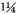hours and is 20°15′ distant;

6. The sixth, which is under the summer tropic, differs by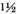hours and is 23°50′ distant, and is drawn through Syene;

7. The seventh differs by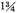hours and is 27°10′ distant;

8. The eighth3 differs by 2 hours and is 30°20′ distant;

9. The ninth differs by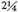hours and is 33°20′ distant;

10. The tenth differs by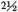hours and is 36° distant, and is drawn through Rhodes;

11. The eleventh differs by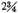hours and is 38°35′ distant;

12. The twelfth4 differs by 3 hours and is 40°55′ distant;

13. The thirteenth differs by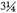hours and is 43°5′ distant;

14. The fourteenth5 differs by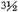hours and is 45° distant;

15. The fifteenth6 differs by 4 hours and is 48°30′ distant;

16. The sixteenth differs by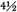hours and is 51°30′ distant;

17. The seventeenth differs by 5 hours and is 54° distant;

18. The eighteenth differs by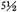hours and is 56°10′ distant;

19. The nineteenth differs by 6 hours and is 58° distant;

20. The twentieth differs by 7 hours and is 61° distant;

21. The twenty-first differs by 8 hours and is 63° distant, and is drawn through Thule.7

Another parallel will be drawn south of the equator and distant one-half hour from it. This parallel, through Cape Rhaptus and Cattigara, will be approximately the same distance in degrees from the equator as is the parallel on the other side, viz., 8°25′.

24. A method for representing the inhabited earth on a plane to correspond to its position on a sphere

I

In drawing a map on a plane surface our method of obtaining the proper proportions of the outermost parallels will be as follows. We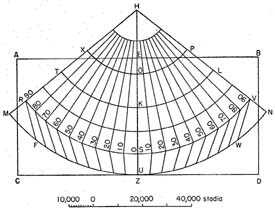shall construct a rectangular table ABDC with side AB approximately double side AC. Let us assume that AB in the upper portion will represent the northern part of the map. Then, bisecting AB with line EZ at right angles to it, we shall apply congruent to AB a straight ruler in such a way that the [perpendicular] line passing through the midpoint of its length forms a single straight line with EZ, that is line EH.1 Let EH contain 34 units and HZ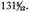. With H as a center and a radius of 79 units along HZ we shall describe an arc TKL representing the parallel through Rhodes.

To obtain the limits of longitude, a distance of six hours on either side of K, we shall take a distance of four units on HZ, the line representing the central meridian, as equal to five units on the parallel through Rhodes, because of the ratio of five to four between the great circle and the parallel through Rhodes.1 Taking 18 such units on either side of K along arc TKL, we shall have the points through which it will be necessary to draw from H the meridians representing intervals of one-third of an hour, including the two boundary meridians HTM and HLN.

The parallel through Thule will accordingly be drawn 52 units distant from H along HZ, viz., XOP, and the equator, similarly, 115 units distant from H, viz., RSV. The southernmost parallel MUN, opposite that through Meroe, will be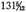units distant from H.

Therefore the ratio between RSV and XOP will be 115 to 52, corresponding to the ratio between these parallels on a sphere, since HS is to HO as 115 to 52, and arc RSV is to arc XOP as HS is to HO.

The meridian distance OK, i.e., the distance from the parallel through Thule to that through Rhodes, will be found to be 27 units; the distance KS, from the parallel through Rhodes to the equator, 36 units; the distance SU, from the equator to the parallel lying opposite that which passes through Meroe,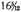units. Again, the distance OU, the breadth of the known portion of the earth, is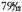or in whole numbers 80 units, and the parallel TKL, the mean longitudinal distance, will contain 144 of these units, which accords with the basic assumptions underlying our demonstrations. For the ratio of the breadth [of our inhabited portion of the world] to its length along the parallel through Rhodes, 40,000 stades to 72,000, is substantially the same.2 Now we shall draw the other parallel circles, if we desire, with H again as center and with radii equal to the distances from S by which these parallels are separated from the equator, as has been set forth.

Now it will be possible for us to draw the lines which represent meridians not all the way to the parallel circle MUN but only as far as the equator RSV, and then, dividing MUN into 180 parts equal in size and number to those on the parallel through Meroe, to draw to the divisions on MUN from the divisions on the equator intermediate straight lines representing the meridians, so that their position on the other side of the equator and sloping toward the south may be indicated by the change in direction, e.g., lines RF and VW.

Furthermore, in order that we may more easily indicate the position of the places to be represented, we shall make a narrow ruler equal in length either to HZ or merely to HS. Fixing this ruler at H as a pole, so that as it turns over the whole length of the map we may carefully apply one of its sides to the straight lines representing the meridians (the side is so cut out as to pass directly through the pole), we shall divide this side into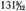units extending over HZ or 115 extending merely over HS, and shall mark the numbers beginning with the mark at the equator. And from these numbers it will be possible also to indicate the parallels, so that it will not be necessary on the map itself to divide the meridian OU into all its parts and to mark the numbers along the divisions. For to do this would make indistinct the names of the places near that meridian,

And so, after dividing the equator, too, into the 180 parts representing the twelve hours, and after indicating the numbers beginning with the meridian furthest to the west, we shall bring the side of the ruler to the required degree of longitude. Then, using the divisions on the ruler we shall arrive at the required position with respect to latitude and make the proper mark in each case, as was pointed out in the case of places on the sphere.

II

But we can make a still truer and better proportioned map of the inhabited earth on a plane surface if we make the meridian lines appear as they do on the surface of a sphere when the axis of vision1 passes through the center of the sphere and that point of the sphere at which the meridian bisecting the length of the known portion of the earth and the parallel bisecting its breadth intersect. In this way the opposite boundaries are symmetrical with respect to the vision and are so perceived.2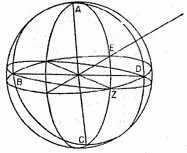Now, in the first place, in order to find the amount of inclination of the parallel circles to the plane that passes through the aforesaid intersection and the center of the sphere and is perpendicular to the central meridian of longitude, let the great circle which forms the boundary of the visible hemisphere be ABCD. And let the semicircle of the meridian which bisects this hemisphere be AEC, and the point of intersection of this semicircle and the parallel that bisects the breadth of the known portion of the earth be the point E, directly in the axis of vision. Now let a semicircle BED of a great circle be drawn through E at right angles to AEC. The plane of this semicircle will clearly include the axis of vision,1 Cutting off arc EZ of 23°50′—for this is the distance from the equator to the parallel through Syene, which parallel approximately bisects the breadth of the inhabited portion of the earth—draw through Z a semicircle, BZD, of the equator. Therefore the plane of the equator and the planes of the other parallels will appear inclined to the plane [BED] which includes the axis of vision by an amount equal to arc EZ, that is, by 23°50′.

Now let the straight lines AEZC and BED be considered as representing arcs, BE bearing to EZ the ratio of 90 to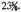With CA produced, let the center about which the arc of circle BZD will be drawn fall at H. And let it be required to find the ratio of HZ to EB. Draw line ZB, bisect it at T, and draw TH which is, of course, perpendicular to BZ.

Since, then, EZ has been assumed to containunits of the kind of which BE contains 90, the hypotenuse BZ will contain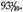Again, angle BZE will contain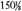units of the kind of which two right angles contain 360, and the remaining angle THZ will contain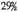such units. Hence the ratio of HZ to ZT is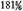to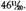And if line TZ contains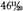units, BE will contain 90 such units. Therefore line HZ will contain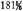units of the kind of which BE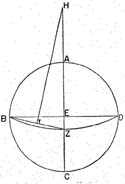contains 90 and ZE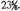And H will be the point from which all the parallels will be drawn on the map made on a plane.

Having found these data, let ABDC [p. 176] be the surface of the map, with AB double AC, AE equal to EB, and EZ perpendicular to AE and EB. Let a line equal to EZ be divided into the 90 parts that represent a quadrant of a circle. If we lay off ZH containing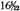such parts, HT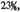and HK 63, and consider H as on the equator, then T will be on the point through which will be drawn the parallel through Syene, approximately bisecting the breadth of the known portion of the earth, Z the point through which will be drawn the parallel forming its southern boundary and lying opposite the parallel through Meroe, and K the point through which will be drawn the parallel forming its northern boundary and passing through the island of Thule.

Now prolonging line ZE and taking HL on that line equal to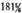units, or merely 180 (for the difference on the map will be negligible), with L as center and radii LZ, LT, and LK, respectively, we shall draw arcs PKR, XTO, and MZN, Thus the proper degree of inclination of the parallel circles to the plane which includes the axis of vision1 will be preserved, since in this case too the axis of vision must be directed toward T and must be perpendicular to the plane of the map so that here too the opposite boundaries of the map may be symmetrically envisioned.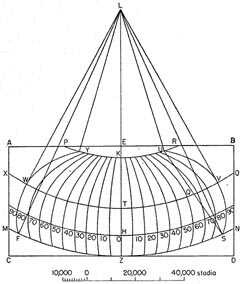Now in order that the length too may properly correspond to the breadth of the inhabited portion of the earth (for on the assumption that the length of the great circle of the sphere is 5 units, the parallel circle through Thule is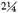units, that through Syene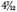units, and that through Meroe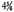units), we must place on either side of the straight line representing meridian ZK eighteen meridians at intervals of one-third of an equinoctial hour to complete the total number of meridian semicircles included within the whole extent of longitude. Thus we shall make divisions on each of the three aforesaid parallels at intervals of five degrees, the number of degrees in one-third of an equinoctial hour.1 In the case of these parallels our divisions from K will be at intervals of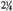units, from T at intervals of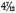units, and from Z at intervals of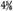units, line EZ being assumed to contain 90 such units.

Then having drawn arcs in each case through the three corresponding points, which will be sufficient to represent all the meridians, among them arcs SVU and FWY which bound the entire extent of longitude, we shall also add arcs to represent the other parallels using as center L and as radii the intervals along ZK corresponding to the distances of the respective parallels from the equator.

It is immediately clear that such a map as we have just described corresponds more closely to the form of a map on a sphere than does the map previously described. For in the case of a sphere too, when it remains unmoved and is not rotated (a necessary condition in the case of a map on a plane), and the axis of vision is directed to the center of the map, only one meridian, the central one, falling in the plane which includes the axis of vision, can appear as a straight line. All the other meridians on either side of this one appear bent concavely towards it, the more so the further they are removed from it. Now this curvature will be preserved to the proper degree in the case of the map on the plane as it was just described. So also will the proper proportion of the parallels of latitude to one another be preserved, not alone in the case of the equator and the parallel through Thule, as in the map previously described, but also, as nearly as possible, in the case of the other parallels, as will be evident upon trial. Again, the proper proportion of the entire latitude to the entire longitude will be preserved, not merely, as in the map previously described, on the parallel drawn through Rhodes, but with substantial accuracy on all the parallels.

For if in this case too, as in the former map, we draw SQU as a straight line, arc TQ will, clearly, bear a smaller ratio to arcs ZS and KU than is the proper ratio on this map, i.e., the ratio that was taken along the whole arc TV, determined according to the equator. And if we make this arc [TQ] the proper length in relation to KZ, the extent of latitude of the known portion of the earth, arcs SZ and KU will be longer than they should properly be in relation to ZK, as indeed arc TV is. If, again, we keep arcs ZS and KU of the proper length to correspond to arc ZK, arc TQ will be smaller than it should be in relation to arc KZ, just as it is smaller than arc TV.

In these respects, therefore, this method has the advantage over that previously described, but is inferior in point of convenience of drawing. For in the case of the map previously described it was possible, by applying and pivoting the ruler, after only one of the parallels had been drawn and divided, to fix the position of every place. In the map just described, however, this easy procedure is not available because of the curvature of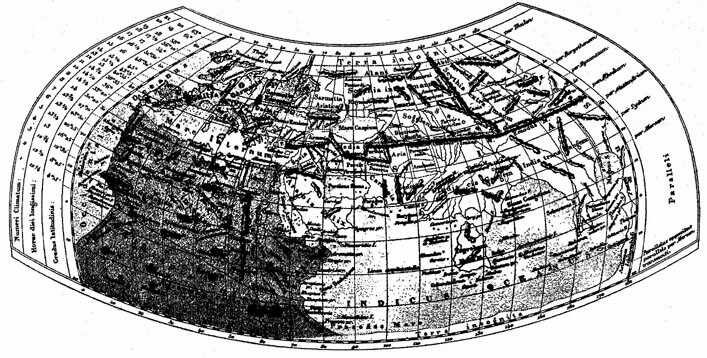Map of the world based on Ptolemy’s description Note the latitude and length of the longest day at the various "climata."

the meridian lines with respect to the central one. It is necessary, therefore, to draw all the arcs and, in the case of positions falling inside the boxed off areas1 to get at them by a calculation of their relation to all four sides enclosing the area in question, a calculation based on the latitude and longitude assigned each such position. This being the case, though both here and everywhere I think that the better and more difficult method is to be more highly regarded than the inferior and easier method, both methods that have been described should still be kept available, for the sake of those who by reason of their unwillingness to exert themselves will have recourse to the easier method.

1 I.e., as on the spherical surface itself. Admittedly a strained interpretation of of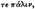the reading of the manuscripts. But Müller’s conjecture,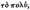is hardly an improvement.

2 I.e., an arc representing 5° of longitude on the parallel through Rhodes is equal in length to an arc representing 4° of latitude, or, what amounts to the same thing, the parallel circle through Rhodes is four-fifths as long as the equator or any great circle on the earth.

3 Perhaps what is meant is that the parallel through Rhodes over the entire length of the inhabited world (180° equal to 144° on a great circle) would be about twice as long as the meridian distance between the parallels through Thule and Cattigara (see pp. 171 f.).

1 In a previous section Ptolemy indicates the total longitude of the inhabited world as approximately 180° (12 hours). Marinus had made this 225° (15 hours). Actually the longitude from the Fortunate Isles to Sera is about 127°.

2 I.e., 16%#186;25′ south latitude. This figure is an approximation to the latitude where the longest day of the year is 13 hours. Meroe itself is about 16°56′ north latitude.

1 I.e., at intervals of 5°.

2 I.e., the longest day of the year at this latitude is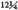hours. In the Almagest Ptolemy gives the procedure for determining the length of the longest day of the year at a given place from the latitude of the place (see p. 84, above; see also H. v.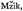op. cit., pp. 90–92). The division of the map by parallels corresponding to the length of the longest day was used by Eratosthenes and, in greater detail, by Hipparchus and Ptolemy. Cf. the system of "climata" in Strabo II. 5.34–43.

3 Some manuscripts add "drawn through Alexandria," though this is probably a later interpolation (see Müiller ad loc.).

4 Some manuscripts add "through the Hellespont."

5 Some manuscripts add "through the middle of Pontus."

6 Some manuscripts add "through the Borysthenes."

7 In the Almagest (II. 6) there are, including the equator, 33 parallels of latitude from 0° to the arctic circle (66°8′40″). The two works show small divergences in the computation of latitudes.

1 In the projection here described the habitable part of the world is represented on a plane made from the surface of a right cone tangent to the original sphere at the parallel through Rhodes (36° north latitude). The vertex is the center from which the parallels of latitude are drawn, while the meridians are straight lines intersecting at the vertex. For a, mathematical treatment of the projection and an explanation of how the numbers are arrived at, see H. v.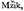op. cit., pp. 93–99.

1 The length of the parallel through Rhodes is four-fifths that of the equator (144:180).

2 I.e.,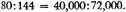The measurement in stades is based on the estimate of 180,000 stades as the circumference of the earth (see p. 150, n. 2, above).

1 I.e., the axis of the cone containing the visual rays.

2 The projection here described is, as Ptolemy points out, more accurate but more difficult than the preceding one. Only one of the meridians—that bisecting the length of the map—is a straight line. For mathematical details see H. v.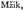op. cit., pp. 100–104. The projections described in this section were not used by Ptolemy in the 26 special maps of the various parts of the oecumene; in these maps the meridians of longitude were drawn parallel to one another and perpendicular to the parallels of latitude.

1 Since it includes two points of this line, E and the center of the sphere.

1 I.e., the plane perpendicular to the surface of the map and determined by the eye and the central meridian.

1 Each of the 36 meridians drawn will be determined by three corresponding points on the parallels.

1 The reference is to the areas cut off between two successive meridian lines and two successive parallel circles represented on the map.

Contents:

### Related Resources

Ptolemy

Title: A Source Book in Greek Science

Select an option:

## Email Options

Title: A Source Book in Greek Science

Select an option: